# Function Machines Worksheet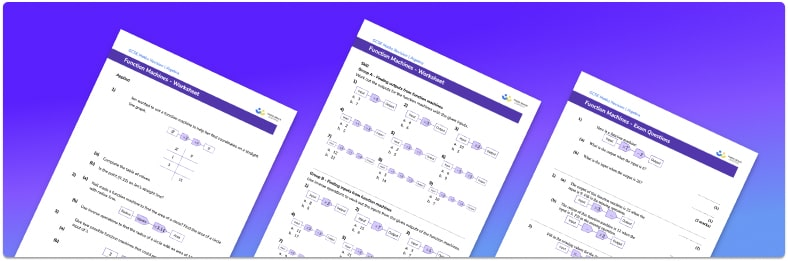• This field is for validation purposes and should be left unchanged.

You can unsubscribe at any time (each email we send will contain an easy way to unsubscribe). To find out more about how we use your data, see our privacy policy.

• Section 1 of the function machines worksheet contains 27 skills-based function machines questions, in 3 groups to support differentiation
• Section 2 contains 4 applied questions with a mix of worded problems and deeper problem solving questions
• Section 3 contains 4 foundation and higher level GCSE exam practice questions to practise your own function machine questions
• Correct answers and a mark scheme for all questions are provided
• Questions follow variation theory with plenty of opportunities for students to work independently at their own level
• All questions created by fully qualified expert secondary maths teachers
• Suitable for GCSE maths revision for AQA, OCR and Edexcel exam boards

### Function machines at a glance

Function machines are a way of representing functions. They are sometimes called number machines and they use an input and an output with various operations to represent a function.

Simple functions can be represented by a one-step function machine where there is only one operation. More complex functions may require two-step function machines and so on.  Function machines are used by inputting a value and performing the stated mathematical operations in order to calculate the output. Function machines can be used in reverse by using inverse operations to work out the input from a given when an output. It is important to have a strong understanding of the order of operations (also known as bidmas) and inverse operations such as knowing the inverse of addition is subtraction and vice-versa.

We can use function machines for solving equations. They work best with simple linear equations but they can also be applied to more complex functions.

Function machines are also linked to different types of graphs including linear and quadratic graphs. Inputting values into the function and recording the solutions in an output table can help to produce the coordinates to plot the graphs.

Looking forward, students can then progress to additional algebra worksheets, for example a simplifying expressions worksheet or simultaneous equations worksheet.For more teaching and learning support on Algebra our GCSE maths lessons provide step by step support for all GCSE maths concepts.

## Related worksheets

Composite and Inverse Functions Worksheet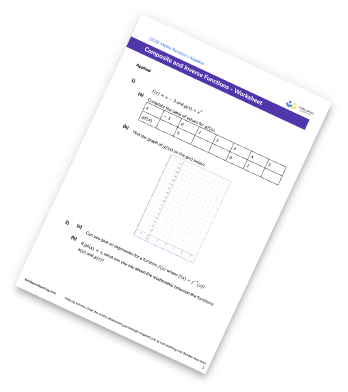Function Notation Worksheet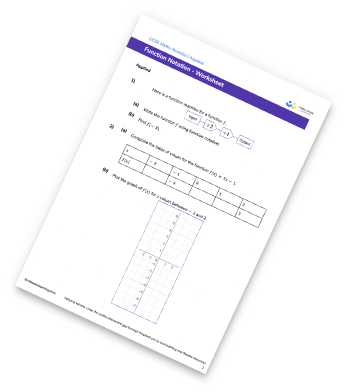Functions Worksheet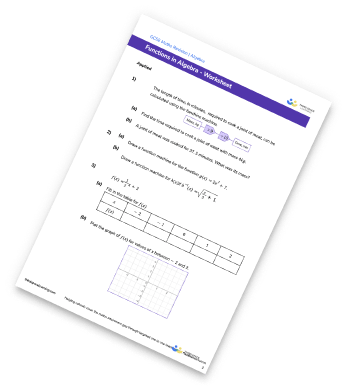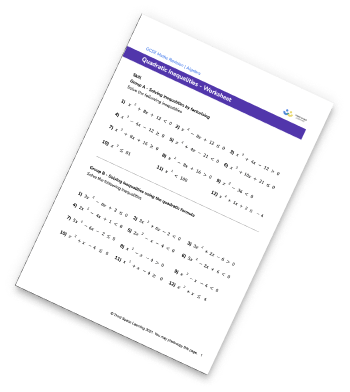## Do you have KS4 students who need more focused attention to succeed at GCSE?There will be students in your class who require individual attention to help them succeed in their maths GCSEs. In a class of 30, it’s not always easy to provide.

Help your students feel confident with exam-style questions and the strategies they’ll need to answer them correctly with our dedicated GCSE maths revision programme.

Lessons are selected to provide support where each student needs it most, and specially-trained GCSE maths tutors adapt the pitch and pace of each lesson. This ensures a personalised revision programme that raises grades and boosts confidence.

Find out more